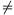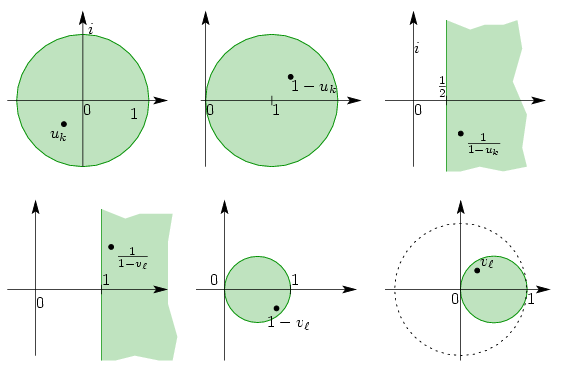Mathematical and Physical Journal
for High Schools
Issued by the MATFUND Foundation
 Already signed up? New to KöMaL?

#Problem A. 430. (May 2007)

A. 430. Let n2 and letbe arbitrary complex numbers with absolute values at most 1 and let

f(x)=(x-u1)(x-u2)...(x-un).

Prove that polynomial f'(x) has a root with a non-negative real part.

(5 pont)

Deadline expired on June 15, 2007.

Solution. If 1 is a multiple root of f then f'(1)=0 and the statement becomes trivial. So we assume that u2,...,un1.

Let the roots of f'(x) be v1,v2...,vn-1, and consider the polynomial g(x)=f(1-x)=a1x+a2x2+...+anxn.

The nonzero roots of g(x) are 1-u2,...,1-un. From the Viéta-formulas we obtainThe roots of polynomial f'(1-x)=-g'(x)=-a1-2a2x-...-nanxn-1 are; from the Viéta formulas again,Combining the two equations,For every k, the number uk lies in the unit disc (or on its boundary), and 1-uk lies in the circle with center 1 and unit radius (or on its boundary). The operation of taking reciprocals can be considered as the combination of an inversion from pole 0 and mirroring over the real axis. Hencelies in the half plane, i.e..

Summing up these inequalities,so at least onelies in the half plane.

Repeating the same geometric steps backwards,### Statistics:

 3 students sent a solution. 5 points: Tomon István. 4 points: Nagy 235 János. Unfair, not evaluated: 1 solutions.

Problems in Mathematics of KöMaL, May 2007